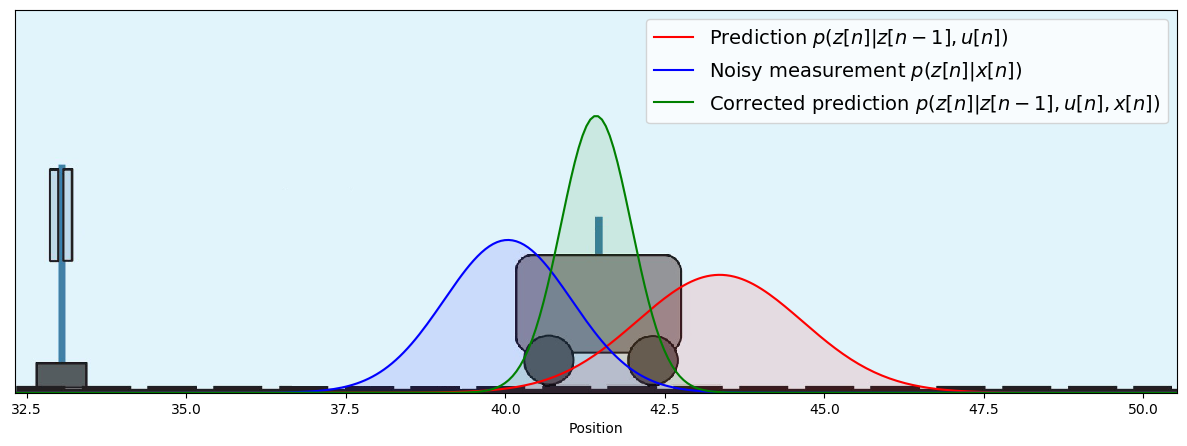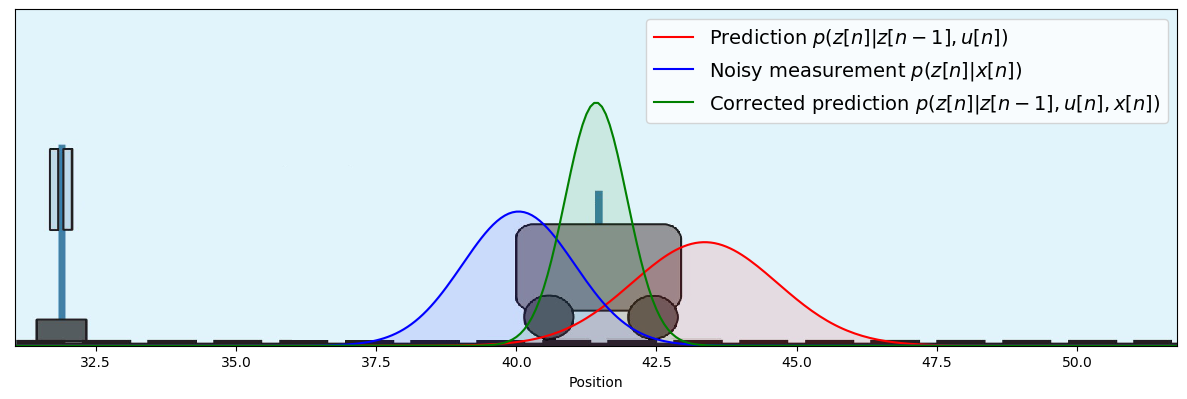# Dynamic Models¶

### Example Problem¶

• We consider a one-dimensional cart position tracking problem, see</span> Faragher 2012.

• The hidden states are the position $z_t$ and velocity $\dot z_t$. We can apply an external acceleration/breaking force $u_t$. (Noisy) observations are represented by $x_t$.

• The equations of motions are given by

\begin{align*} \begin{bmatrix} z_t \\ \dot{z_t}\end{bmatrix} &= \begin{bmatrix} 1 & \Delta t \\ 0 & 1\end{bmatrix} \begin{bmatrix} z_{t-1} \\ \dot z_{t-1}\end{bmatrix} + \begin{bmatrix} (\Delta t)^2/2 \\ \Delta t\end{bmatrix} u_t + \mathcal{N}(0,\Sigma_z) \\ x_t &= \begin{bmatrix} z_t \\ \dot{z_t}\end{bmatrix} + \mathcal{N}(0,\Sigma_x) \end{align*}
• Infer the position $z_t$ after 10 time steps. (Solution later in this lesson).### Dynamical Models¶

• Consider the ordered observation sequence $x^T \triangleq \left(x_1,x_2,\ldots,x_T\right)$.
• (For brevity, we use the notation $x_t^T$ to denote $(x_t,x_{t+1},\ldots,x_T)$ and drop the subscript if $t=1$).
• We wish to develop a generative model $$p( x^T \,|\, \theta)$$ that 'explains' the time series $x^T$.
• We cannot use the IID assumption $p( x^T | \theta) = \prod_t p(x_t \,|\, \theta)$. In general, we can use the chain rule) (a.k.a. the general product rule)
\begin{align*} p(x^T) &= p(x_T|x^{T-1}) \,p(x^{T-1}) \\ &= p(x_T|x^{T-1}) \,p(x_{T-1}|x^{T-2}) \cdots p(x_2|x_1)\,p(x_1) \\ &= p(x_1)\prod_{t=2}^T p(x_t\,|\,x^{t-1}) \end{align*}
• Generally, we will want to limit the depth of dependencies on previous observations. For example, the $M$th-order linear Auto-Regressive (AR) model \begin{align*} p(x_t\,|\,x^{t-1}) = \mathcal{N}\left(x_t \,\middle|\, \sum_{m=1}^M a_m x_{t-m}\,,\sigma^2\,\right) \end{align*} limits the dependencies to the past $M$ samples.

### State-space Models¶

• A limitation of AR models is that they need a lot of parameters in order to create a flexible model. E.g., if $x_t$ is an $K$-dimensional discrete variable, then an $M$th-order AR model will have about $K^{M}$ parameters.
• Similar to our work on Gaussian Mixture models, we can create a flexible dynamic system by introducing latent (unobserved) variables $z^T \triangleq \left(z_1,z_2,\dots,z_T\right)$ (one $z_t$ for each observation $x_t$). In dynamic systems, $z_t$ are called state variables.
• A general state space model is defined by \begin{align*} p(x^T,z^T) &= \underbrace{p(z_1)}_{\text{initial state}} \prod_{t=2}^T \underbrace{p(z_t\,|\,z^{t-1})}_{\text{state transitions}}\,\prod_{t=1}^T \underbrace{p(x_t\,|\,z_t)}_{\text{observations}} \end{align*}
• A common assumption is to let state transitions be ruled by a first-order Markov chain as
$$p(z_t\,|\,z^{t-1}) = p(z_t\,|\,z_{t-1})$$
• In a Markovian state-space model, the observation sequence $x^T$ is not a first-order Markov chain, i.e., for the state-space model \begin{align*} p(x^T,z^T) &= p(z_1) \prod_{t=2}^T p(z_t\,|\,z_{t-1})\,\prod_{t=1}^T p(x_t\,|\,z_t) \end{align*} the following statement holds: $$p(x_t\,|\,x_{t-1},x_{t-2}) \neq p(x_t\,|\,x_{t-1})\,.$$ In other words, the latent variables $z_t$ represent a memory bank for past observations beyond $t-1$. (Proof as exercise).
• The Forney-style factor graph for a state-space model:### Hidden Markov Models and Linear Dynamical Systems¶

• A Hidden Markov Model (HMM) is a specific state-space model with discrete-valued state variables $Z_t$.
• E.g., $Z_t$ is a $K$-dimensional hidden binary 'class indicator' with transition probabilities $A_{jk} \triangleq p(z_{tk}=1\,|\,z_{t-1,j}=1)$, or equivalently $$p(z_t|z_{t-1}) = \prod_{k=1}^K \prod_{j=1}^K A_{jk}^{z_{t-1,j}z_{tk}}$$ which is usually accompanied by an initial state distribution $\pi_k \triangleq p(z_{1k}=1)$.
• The classical HMM has also discrete-valued observations but in pratice any (probabilistic) observation model $p(x_t|z_t)$ may be coupled to the hidden Markov chain.
• Another well-known state-space model with continuous-valued state variables $Z_t$ is the (Linear) Gaussian Dynamical System (LGDS), which is defined as
\begin{align*} p(z_t\,|\,z_{t-1}) &= \mathcal{N}\left(\, A z_{t-1}\,,\,\Sigma_z\,\right) \\ p(x_t\,|\,z_t) &= \mathcal{N}\left(\, C z_t\,,\,\Sigma_x\,\right) \\ p(z_1) &= \mathcal{N}\left(\, \mu_1\,,\,\Sigma_1\,\right) \end{align*}
• Note that the joint distribution over $\{(x_1,z_1),\ldots,(x_t,z_t)\}$ is a (large-dimensional) Gaussian distribution. This means that, in principle, every inference problem on the LGDS model also leads to a Gaussian distribution.
• HMM's and LGDS's (and variants thereof) are at the basis of a wide range of complex information processing systems, such as speech and language recognition, robotics and automatic car navigation, and even processing of DNA sequences.

### Kalman Filtering¶

• Technically, a Kalman filter is the solution to the recursive estimation (inference) of the hidden state $z_t$ based on past observations in an LGDS, i.e., Kalman filtering solves the problem $p(z_t\,|\,x^t)$ based on the previous estimate $p(z_{t-1}\,|\,x^{t-1})$ and a new observation $x_t$ (in the context of the given model specification of course).
• Let's infer the Kalman filter for a scalar linear Gaussian dynamical system: \begin{align*} p(z_t\,|\,z_{t-1}) &= \mathcal{N}(z_t\,|\,a z_{t-1},\sigma_z^2) \tag{state transition} \\ p(x_t\,|\,z_t) &= \mathcal{N}(x_t\,|\,c z_t,\sigma_x^2) \tag{observation} \end{align*}
• Kalman filtering comprises inferring $p(z_t\,|\,x^t)$ from a given prior estimate $p(z_{t-1}\,|\,x^{t-1})$ and a new observation $x_t$. Let us assume that \begin{align} p(z_{t-1}\,|\,x^{t-1}) = \mathcal{N}(z_{t-1} \,|\, \mu_{t-1}, \sigma_{t-1}^2) \tag{prior} \end{align}
• Note that everything is Gaussian, so this is in principle possible to execute inference problems analytically and the result will be a Gaussian posterior:
\begin{align*} \underbrace{p(z_t\,|\,x^t)}_{\text{posterior}} &= p(z_t\,|\,x_t,x^{t-1}) \propto p(x_t,z_t\,|\,x^{t-1}) \\ &\propto p(x_t\,|\,z_t) \,p(z_t\,|\,x^{t-1}) \\ &= p(x_t\,|\,z_t) \, \sum_{z_{t-1}} p(z_t,z_{t-1}\,|\,x^{t-1}) \\ &= \underbrace{p(x_t\,|\,z_t)}_{\text{observation}} \, \sum_{z_{t-1}} \underbrace{p(z_t\,|\,z_{t-1})}_{\text{state transition}} \, \underbrace{p(z_{t-1}\,|\,x^{t-1})}_{\text{prior}} \\ &= \mathcal{N}(x_t\,|\,c z_t,\sigma_x^2) \sum_{z_{t-1}} \mathcal{N}(z_t\,|\,a z_{t-1},\sigma_z^2) \, \mathcal{N}(z_{t-1} \,|\, \mu_{t-1}, \sigma_{t-1}^2) \\ &= c\,\mathcal{N}\left(z_t\bigm| \frac{x_t}{c} ,\left(\frac{\sigma_x}{c}\right)^2\right) \sum_{z_{t-1}} a\,\underbrace{\mathcal{N}\left(z_{t-1}\bigm| \frac{z_t}{a},\left(\frac{\sigma_z}{a}\right)^2 \right) \, \mathcal{N}(z_{t-1} \,|\, \mu_{t-1}, \sigma_{t-1}^2)}_{\text{use Gaussian multiplication formula SRG-6}} \\ &\propto \underbrace{\mathcal{N}\left(z_t\,\bigm| \,\frac{x_t}{c} ,\left(\frac{\sigma_x}{c}\right)^2\right) \cdot \mathcal{N}\left(z_t\, \bigm|\,a \mu_{t-1},\sigma_z^2 + \left(a \sigma_{t-1}\right)^2 \right)}_{\text{use SRG-6 again}} \\ &\propto \mathcal{N}\left( z_t \,|\, \mu_t, \sigma_t^2\right) \end{align*}

with \begin{align*} \rho_t^2 &= \sigma_z^2 + a^2 \sigma_{t-1}^2 \tag{auxiliary variable}\\ K_t &= \frac{c \rho_t^2}{c^2 \rho_t^2 + \sigma_x^2} \tag{'Kalman gain'} \\ \mu_t &= \underbrace{a \mu_{t-1}}_{\text{prior prediction}} + K_t \cdot \underbrace{\left( x_t - c a \mu_{t-1}\right)}_{\text{prediction error}} \tag{posterior mean}\\ \sigma_t^2 &= \left( 1 - K_t \right) \rho_t^2 \tag{posterior variance} \end{align*}

• Kalman filtering consists of computing/updating these last four equations for each new observation ($x_t$).

### Kalman Filtering and the Cart Position Tracking Example Revisited¶

• The Kalman filter equations can also be derived for multidimensional state-space models. In particular, for the model \begin{align*} z_t &= A z_{t-1} + \mathcal{N}(0,\Gamma) \\ x_t &= C z_t + \mathcal{N}(0,\Sigma) \end{align*} the Kalman filter update equations are given by (see Bishop, pg.639) \begin{align*} P_t &= A V_{t-1} A^T + \Gamma &&\text{auxiliary variable}\\ K_t &= P_t C^T \cdot \left( \Sigma + C P_t C^T\right)^{-1} &&\text{Kalman gain vector} \\ \mu_t &= A \mu_{t-1} + K_t\cdot\left(x_t - C A \mu_{t-1} \right) &&\text{posterior state mean}\\ V_t &= \left(I-K_t C \right) V_{t-1} &&\text{posterior state variance} \end{align*}

#### CODE EXAMPLE¶

• We can now solve the cart tracking problem of the introductory example by implementing the Kalman filter.
In :
using Pkg;Pkg.activate("probprog/workspace/");Pkg.instantiate()
using LinearAlgebra, PyPlot
include("scripts/cart_tracking_helpers.jl")

# Specify the model parameters
Δt = 1.0                     # assume the time steps to be equal in size
A = [1.0 Δt;
0.0 1.0]
b = [0.5*Δt^2; Δt]
Σz = convert(Matrix,Diagonal([0.2*Δt; 0.1*Δt])) # process noise covariance
Σx = convert(Matrix,Diagonal([1.0; 2.0]))     # observation noise covariance;

# Generate noisy observations
n = 10                # perform 10 timesteps
z_start = [10.0; 2.0] # initial state
u = 0.2 * ones(n)     # constant input u
noisy_x = generateNoisyMeasurements(z_start, u, A, b, Σz, Σx);

m_z = noisy_x                                    # initial predictive mean
V_z = A * (1e8*Diagonal(I,2) * A') + Σz             # initial predictive covariance

for t = 2:n
global m_z, V_z, m_pred_z, V_pred_z
#predict
m_pred_z = A * m_z + b * u[t]                   # predictive mean
V_pred_z = A * V_z * A' + Σz                    # predictive covariance
#update
gain = V_pred_z * inv(V_pred_z + Σx)            # Kalman gain
m_z = m_pred_z + gain * (noisy_x[t] - m_pred_z) # posterior mean update
V_z = (Diagonal(I,2)-gain)*V_pred_z             # posterior covariance update
end
println("Prediction: ",ProbabilityDistribution(Multivariate,GaussianMeanVariance,m=m_pred_z,v=V_pred_z))
println("Measurement: ", ProbabilityDistribution(Multivariate,GaussianMeanVariance,m=noisy_x[n],v=Σx))
println("Posterior: ", ProbabilityDistribution(Multivariate,GaussianMeanVariance,m=m_z,v=V_z))
plotCartPrediction2(m_pred_z, V_pred_z, m_z, V_z, noisy_x[n], Σx);

Activating environment at ~/Documents/biaslab/repos/BMLIP/lessons/notebooks/probprog/workspace/Project.toml
Prediction: 𝒩(m=[43.36, 4.58], v=[[1.30, 0.39][0.39, 0.34]])Measurement: 𝒩(m=[40.04, 3.18], v=[[1.00, 0.00][0.00, 2.00]])

Posterior: 𝒩(m=[41.42, 3.91], v=[[0.55, 0.15][0.15, 0.24]])



### Recap Dynamical Models¶

• Dynamical systems do not obey the sample-by-sample independence assumption, but still can be specified, and state and parameter estimation equations can be solved by similar tools as for static models.
• Two of the more famous and powerful models with latent states include the hidden Markov model (with discrete states) and the Linear Gaussian dynamical system (with continuous states).
• For the LGDS, the Kalman filter is a well-known recursive state estimation procedure. The Kalman filter can be derived through Bayesian update rules on Gaussian distributions.
• If anything changes in the model, e.g., the state noise is not Gaussian, then you have to re-derive the inference equations again from scratch and it may not lead to an analytically pleasing answer.
• $\Rightarrow$ Generally, we will want to automate the inference processes. This topic is the domain of Probabilistic Programming, whih we will discuss later in this course.

# OPTIONAL SLIDES¶

### Extensions of Generative Gaussian Models¶

• Using the methods of the previous lessons, it is possible to create your own new models based on stacking Gaussian and categorical distributions in new ways:### Message Passing in State-space Models¶

• As we have seen, inference tasks in linear Gaussian state space models can be analytically solved, see e.g. Faragher, 2012 and the Kalman filter derivation above.
• However, these derivations quickly become cumbersome and prone to errors.
• Alternatively, we could specify the generative model in a (Forney-style) factor graph and use automated message passing to infer the posterior over the hidden variables. E.g., the message passing schedule for Kalman filtering looks like this:### The Cart Tracking Problem Revisited: Inference by Message Passing¶

• Let's solve the cart tracking problem by sum-product message passing in a factor graph like the one depicted above. All we have to do is create factor nodes for the state-transition model $p(z_t|z_{t-1})$ and the observation model $p(x_t|z_t)$. Then we just build the factor graph and let ForneyLab execute the message passing schedule.

#### CODE EXAMPLE¶

• We'll implement the following model using ForneyLab:
\begin{align*} \begin{bmatrix} z_t \\ \dot{z_t}\end{bmatrix} &= \begin{bmatrix} 1 & \Delta t \\ 0 & 1\end{bmatrix} \begin{bmatrix} z_{t-1} \\ \dot z_{t-1}\end{bmatrix} + \begin{bmatrix} (\Delta t)^2/2 \\ \Delta t\end{bmatrix} u_t + \mathcal{N}(0,\Sigma_z) \\ \mathbf{x}_t &= \begin{bmatrix} z_t \\ \dot{z_t}\end{bmatrix} + \mathcal{N}(0,\Sigma_x) \end{align*}

Since the factor graph is just a concatination of $n$ identical "sections" (one for each time step), we only have to specify a single section. When running the message passing algorithm we will explictly use the posterior of the previous timestep as prior in the next one. Let's build a section of the factor graph:

In :
fg = FactorGraph()
z_prev_m = Variable(id=:z_prev_m); placeholder(z_prev_m, :z_prev_m, dims=(2,))
z_prev_v = Variable(id=:z_prev_v); placeholder(z_prev_v, :z_prev_v, dims=(2,2))
bu = Variable(id=:bu); placeholder(bu, :bu, dims=(2,))

@RV z_prev ~ GaussianMeanVariance(z_prev_m, z_prev_v, id=:z_prev) # p(z_prev)
@RV noise_z ~ GaussianMeanVariance(constant(zeros(2), id=:noise_z_m), constant(Σz, id=:noise_z_v)) # process noise
@RV z = constant(A, id=:A) * z_prev + bu + noise_z; z.id = :z # p(z|z_prev) (state transition model)
@RV x ~ GaussianMeanVariance(z, constant(Σx, id=:Σx)) # p(x|z) (observation model)
placeholder(x, :x, dims=(2,));
ForneyLab.draw(fg)


Now that we've built the factor graph, we can perform Kalman filtering by inserting measurement data into the factor graph and performing message passing.

In :
include("scripts/cart_tracking_helpers.jl")
eval(Meta.parse(sumProductAlgorithm(z))) # build message passing algorithm
marginals = Dict()
messages = Array{Message}(undef,6)
z_prev_m_0 = noisy_x
z_prev_v_0 = A * (1e8*Diagonal(I,2) * A') + Σz
for t=2:n
data = Dict(:x => noisy_x[t], :bu => b*u[t],:z_prev_m => z_prev_m_0, :z_prev_v => z_prev_v_0)
step!(data, marginals, messages) # perform msg passing (single timestep)
# Posterior of z becomes prior of z in the next timestep:
z_prev_m_0 = ForneyLab.unsafeMean(marginals[:z])
z_prev_v_0 = ForneyLab.unsafeCov(marginals[:z])
end
# Collect prediction p(z[n]|z[n-1]), measurement p(z[n]|x[n]), corrected prediction p(z[n]|z[n-1],x[n])
prediction      = messages.dist # the message index is found by manual inspection of the schedule
measurement     = messages.dist
corr_prediction = convert(ProbabilityDistribution{Multivariate, GaussianMeanVariance}, marginals[:z])
println("Prediction: ",prediction)
println("Measurement: ",measurement)
println("Posterior: ", corr_prediction)

# Make a fancy plot of the prediction, noisy measurement, and corrected prediction after n timesteps
plotCartPrediction(prediction, measurement, corr_prediction);Prediction: 𝒩(m=[43.36, 4.58], v=[[1.30, 0.39][0.39, 0.34]])

Measurement: 𝒩(m=[40.04, 3.18], v=[[1.00, 0.00][0.00, 2.00]])

Posterior: 𝒩(m=[41.42, 3.91], v=[[0.55, 0.15][0.15, 0.24]])


• Note that both the analytical Kalman filtering solution and the message passing solution lead to the same results. The advantage of message passing-based inference with ForneyLab is that we did not need to derive any inference equations. ForneyLab took care of all that.
• ForneyLab is an example of a probabilistic programming language (PPL). More about PPLs later in the PP minicourse.
In :
open("../../styles/aipstyle.html") do f display("text/html", read(f, String)) end

In [ ]: